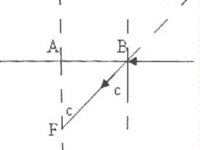## Sunday, November 12, 2006

### Additional MCQ on Refraction at Plane SurfacesIn continuation of the post dated 11th November 2006, here is another MCQ which appeared in the Kerala Medical Entrance 2005 test paper:
A fish looking from within water sees the outside world through a circular horizon. If the fish is √7 m below the surface of water, what will be the radius of the circular horizon?
(a) 3m (b) 3/√7m (c) √7m (d) 3√7m (e) 4m
Note that refractive index of water is not given. You are expected to remember it. Most of you know it as 1.33. On many occasions it will be useful to remember the value as 4/3. As you can see from the figure, the fish at F can see the outside world through a cone of semi angle equal to the critical angle ‘c’ of water because the rays of light at grazing incidence are refracted in to water at critical angle ‘c’. The circular horizon meant in the question has radius AB which is equal to √7 tan c. We have sin c = 1/n = 1/(4/3) =3/4. To obtain tan c, you may imagine a right angled triangle having opposite side 3 and hypotenuse 4 to obtain the adjacent side √7 so that you get tan c = 3/√7.
Since the radius of the circular horizon is √7 tan c,
you get the answer as 3m.
Angle of a prism is ‘A’ and its one surface is silvered. Light rays falling at an angle of incidence 2A on first surface return back through the same path after suffering reflection at the second silvered surface. Refractive index of the material is
(a) 2sinA (b) 2cosA (c) 1/2cosA (d) tanA
Since the ray retraces its path on falling (at D) on the silvered face AC of the prism, it follows that it is incident normally on this face. The angle of refraction (r) at the first face is shown in the figure. From the triangle NAD, angle DNA = 90-A. Therefore, r = A. The refractive index of the material of the prism is therefore given by n = sini/sinr = sin(2A)/ sinA = 2sinA cosA/sinA = 2cosA.
The following MCQ appeared in E.A.M.C.E.T. (Medical) Andhra Pradesh-2003 question paper:
A prism of refractive index μ and angle A is placed in the minimum deviation position. If the angle of minimum deviation is A, then the value of A in terms of μ is
(a) sin-1(μ/2) (b) sin-1 √[(μ-1)/2] (c) 2cos-1(μ/2) (d) cos-1(μ/2)
In the minimum deviation position, we have μ = sin[(A+D)/2] / sin(A/2) where D is the minimum deviation. Putting D = A, as given in the question, we obtain μ = sinA/sin(A/2) = [2sin(A/2)cos(A/2)]/sin(A/2) = 2cos(A/2). Therefore, cos(A/2) = μ/2, from which A = 2cos-1 (μ/2).
Here is an interesting question. It is simple, but you may have many doubts on the method of solving it:
One face A of a glass slab of thickness ‘t’ is silvered. An ink mark is made on the opposite face B. If you look through the face B, what is the distance between the ink mark and its image formed by reflection at the silvered face? (Refractive index of glass is μ).
(a) 2t (b) t + (t/μ) (c) t(1+μ) (d) 2μt (e) 2t/μ
You can work it out from first principles using laws of reflection and refraction and of course geometry and trigonometry and get the correct option which is (e). But you will waste a lot of time in the process. Better, do it as follows:
When you look through the face B, the silvered face A (which is at the real distance ‘t’ from B which carries the ink spot) will appear to be at the apparent distance t/μ from B (and the ink spot). The reflected image of the ink spot is at the same distance t/μ behind the apparent silvered face. So, the distance between the ink spot and its reflected image will be (t/μ + t/μ) = 2t/μ.

1.Anonymous4:27 PM

Sir,
I am a student from Delhi preparing for IITJEE and oder entrances..Cud u plz show some light on JEE 2007 pattern and how they are goin to fit 3 subjects in 2 paperz..Also, the collection of Q's here is indeed very gud..I'll be a regular visitor here..
Rahul

2.There is no need of worrying about the pattern. Of course you will come to know about it in due course. Remember that those who are responsible for conducting the exam are in fact trying to make it pattern independent (and coaching class independent as far as possible!). So study the subject well and you won't have to worry about the outcome.
A visit to www.rediff.com/money/2005/oct/11inter.htm may be helpful to you.

3.Anonymous8:06 PM

sir im a 11 student ..promotes to 12 now.. im a keralite..i wud lik to know that how should i prepare for clearing all india medical entrance with high score..which all books,magazines should i refer..?

4.NCERT text books are good for the preparation. You will find a link to the NCERT online text books on the left of this page under 'Other Resource Links'. Go through old AIPMT questions. You will find many solved questions on this site as well as at entrancephysics.blogspot.com.